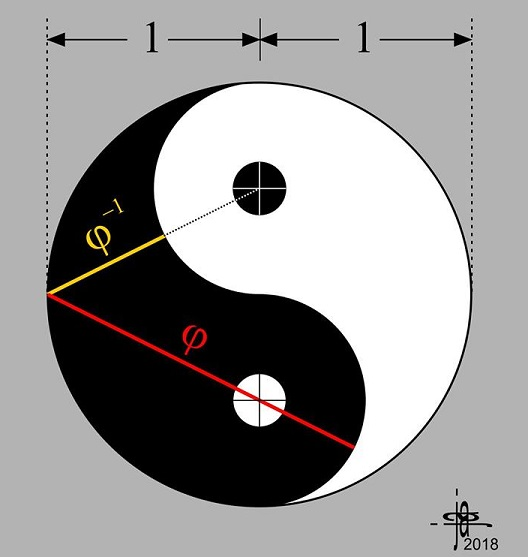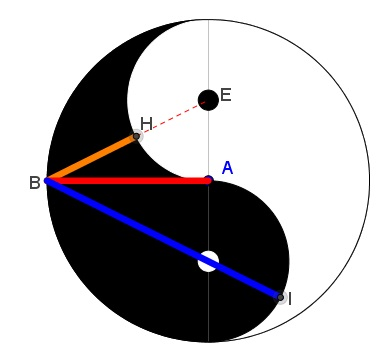# Golden Ratio in Yin-Yang

### Problem### Another Variant### Solution

We refer to the diagram below:Assuming, $AB=1,$ $\displaystyle AE=\frac{1}{2}$ and $\displaystyle BE=\frac{\sqrt{5}}{2}.$ It follows that

$\displaystyle BI=\frac{\sqrt{5}}{2}+\frac{1}{2}=\varphi$

and

$\displaystyle BH=\frac{\sqrt{5}}{2}-\frac{1}{2}=\varphi^{-1}.$

### Acknowledgment

John Arioni has kindly posted at the CutTheKnotMath facebook page his discovery of the Golden Ratio in the famous Yin-Yang diagram. This is of course true that where there is $\varphi$ there is also $\varphi^{-1}.$ But in no other place pointing out both of them is more appropriate than in the Yin-Yang.

John has intimated that he was led to finding the Golden Ratio in Yin-Yang by his previous discoveryIndependently, Yin-Yang conceals another occurrence of the Golden Ratio.

Also, John has eventually found an additional occurrence of the Golden Ratio in the diagram:### Golden Ratio